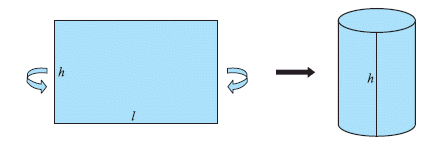Email us to get an instant 20% discount on highly effective K-12 Math & English kwizNET Programs!

#### Online Quiz (WorksheetABCD)

Questions Per Quiz = 2 4 6 8 10

### Geometry6.17 Curved Surface Area of a Cylinder

 If you take a rectangular sheet of paper with length l and height h and connect the two ends you will get a cylinder.The area of the sheet gives the curved surface area of the cylinder. The length of the sheet is equal to the circumference of the circular base which is equal to 2*pi*r. So curved surface area of the cylinder = area of the rectangular sheet = length x width = perimeter of the base of the cylinder x h Curved surface area = 2 * pi * r * h.Example: The ratio of the radius and height of a cylinder is 5:3. The radius is 25cm. Find its curved surface area. Solution: Given that, r = 25cm. Height (h) = 25 * 3/5 = 15cm. Curved surface area = 2 * pi * r * h. Here, r = 25cm. and h = 15cm. = 2 * 22/7 * 25 * 15 = 2357.14 Sq. cm. Directions: Solve the following problems. Also write at least 5 examples of your own.